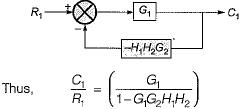Courses

# Test: Block Diagrams

## 10 Questions MCQ Test Control Systems | Test: Block Diagrams

Description
This mock test of Test: Block Diagrams for Electrical Engineering (EE) helps you for every Electrical Engineering (EE) entrance exam. This contains 10 Multiple Choice Questions for Electrical Engineering (EE) Test: Block Diagrams (mcq) to study with solutions a complete question bank. The solved questions answers in this Test: Block Diagrams quiz give you a good mix of easy questions and tough questions. Electrical Engineering (EE) students definitely take this Test: Block Diagrams exercise for a better result in the exam. You can find other Test: Block Diagrams extra questions, long questions & short questions for Electrical Engineering (EE) on EduRev as well by searching above.
QUESTION: 1

### The transfer function of the control system represented by the block diagram shown below is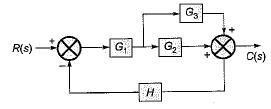Solution:

On combining the blocks G2 and G3 which are in parallel, the given block diagram will be reduced as shown below.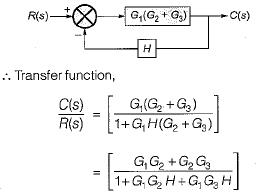QUESTION: 2

### Which one of the following block diagrams in options given is equivalent to the below shown block diagram?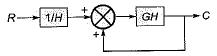Solution:

The T.F. of the given block diagram can be reduced to: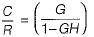Its block diagram is shown below, where a closed loop feedback system is transformed into an unity feedback system and vice-versa,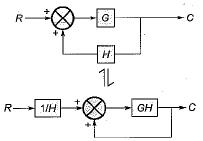QUESTION: 3

### Consider the following statements: 1. Transfer function is an expression which relates output to input in s-domain. 2. Transfer function gives information about the internal structure of the system. 3. A system can be represented by the block diagram if the transfer function of the system is known. 4. Block diagram is the flow of system variables from one block to another block represented by a single line. Q. Which of the above statements is/are true?

Solution:

Transfer function does not gives information about the internal structure of the system. Hence, staement-2 is false.

QUESTION: 4

The ratio C(s)/R(s) for the system shown in figure below is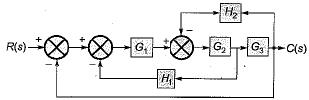Solution:

On shifting the take-off point beyond the block G3 and combining blocks G2 and G3, the given block diagram is reduced as shown below.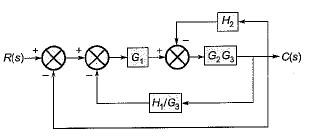The block diagram is further reduced as shown below.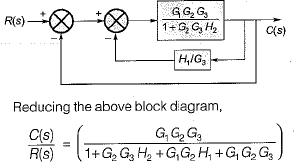QUESTION: 5

The transfer function C(s)/R(s) for the system described by the block diagram shown below is given by: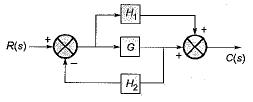Solution:

On shifting the take-off point beyond block G, we have the reduced block diagram as shown below: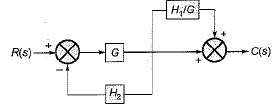On further reducing the above block diagram, we get the block diagram as shown below.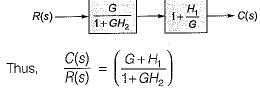QUESTION: 6

The transfer matrix for the multi input-multi output (MIMO) system represented by the block diagram shown below is given by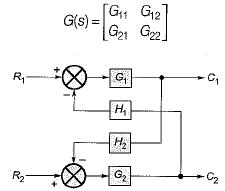Match List-I with List-II and select the correct answer using the codes given below the lists: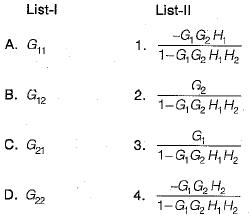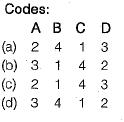Solution: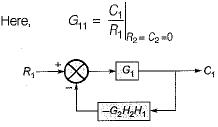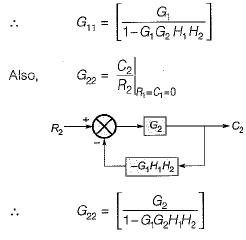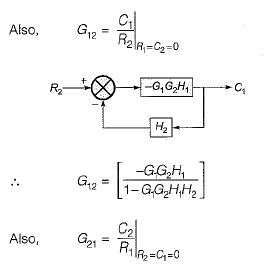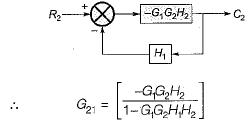QUESTION: 7

Consider the three block diagrams A, B and C shown below.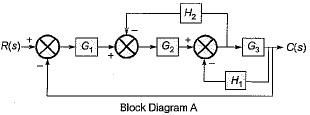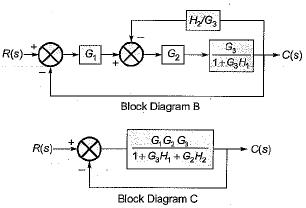Q. Which one of the following statements is correct in respect of the above block diagrams?

Solution:

Shifting the take-off point after block G3 in block diagram-A, the reduced block diagram will be as shown below.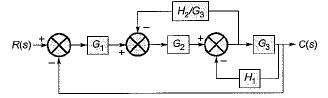Reducing further, the block diagram is reduced as shown below.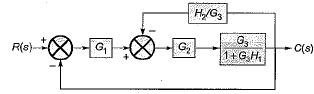Thus, the block diagrams A and B are equivalent to each other.
On further reducing the block diagram-B, we get the block diagram-C. Hence, all the three block diagrams A, B and C are equivalent to each other,

QUESTION: 8

The poles of the transfer function C(s)/R(s) of the system represented by the block diagram shown below are located at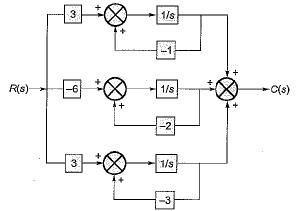Solution:

The given block diagram can be reduced as shown below.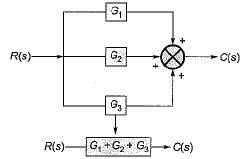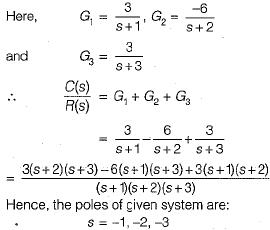QUESTION: 9

The transfer function C(s) / R(s) for the block diagram shown below is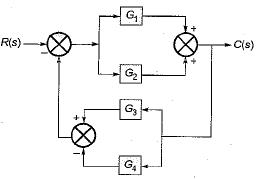Solution:

Combining the blocks in parallel, the block diagram is reduced as shown below.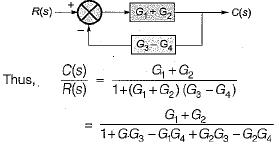QUESTION: 10

The value of for the system described by the block diagram shown in figure below Is.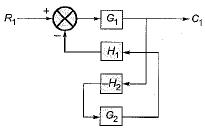Solution:

The given block diagram can be reduced as shown below.